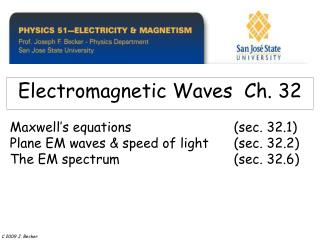# Electromagnetic Waves Ch. 32 - PowerPoint PPT PresentationDownload PresentationElectromagnetic Waves Ch. 32

Electromagnetic Waves Ch. 32
Download Presentation## Electromagnetic Waves Ch. 32

- - - - - - - - - - - - - - - - - - - - - - - - - - - E N D - - - - - - - - - - - - - - - - - - - - - - - - - - -
##### Presentation Transcript

1. Electromagnetic Waves Ch. 32 Maxwell’s equations (sec. 32.1) Plane EM waves & speed of light (sec. 32.2) The EM spectrum (sec. 32.6) C 2009 J. Becker

2. MAXWELL’S EQUATIONS The relationships between electric and magnetic fields and their sources can be stated compactly in four equations, called Maxwell’s equations. Together they form a complete basis for the relation of E and B fields to their sources. C 2004 Pearson Educational / Addison Wesley

3. A capacitor being charged by a current ic has a displacement current equal to iC between the plates, with displacement current iD = e A dE/dt. This changing E field can be regarded as the source of the magnetic field between the plates.

4. A capacitor being charged by a current iC has a displacement current equal to iC in magnitude between the plates, with DISPLACEMENT CURRENT iD = e A dE/dt From C = e A / d and DV = E d wecan useq = C V to get q = (e A / d) (E d ) = e E A = e F E and from iC = dq / dt = e A dE / dt = e dF E / dt= iD We have now seen that achanging E field can produce a Bfieldand from Faraday’s Law achanging B field can produce an E field (or emf) C 2009 J. Becker

5. MAXWELL’S EQUATIONS The relationships between electric and magnetic fields and their sources can be stated compactly in four equations, called Maxwell’s equations. Together they form a complete basis for the relation of E and B fields to their sources. C 2004 Pearson Educational / Addison Wesley

6. An electromagnetic wave front. The plane representing the wave front (yellow) moves to the right with speed c. The E and B fields are uniform over the region behind the wave front but are zero everywhere in front of it.

7. Gaussian surface for a plane electromagnetic wave. The total electric flux and total magnetic flux through the surface are both zero.

8. Applying Faraday’s law to a plane wave. • òEdl= - d FB /dt • 1.òEo dl= -Ea (cos 90o = 0) • In time dt the wave front moves to the right a distance c dt. The magnetic flux through the rectangle in the xy-plane increases by an amount d FB equal to the flux through the shadedrectangle in the xy-plane with area ac dt, that is, d FB = Bac dt. So-d FB /dt = -Bac and E = Bc

9. Applying Ampere’s law to a plane wave. òBdl= mo eod FE /dt 1. ò Bo dl= Ba (cos 90o = 0) 2. In time dt the wave front moves to the right a distance c dt. The electric flux through the rectangle in the xz-plane increases by an amount d FE equal to E times the area ac dt of the shaded rectangle, that is, d FE = Eac dt. Thus d FE/dt = Eac. Ba = mo eo Eac B = mo eo E c and fromE = Bc and B = mo eo E cWe must have c = 1 / (mo eo)1/2 c = 3.00 (10)8 m/sec

10. Faraday’s law applied to a rectangle with height a and width Dx parallel to the xy-plane.

11. Ampere’s law applied to a rectangle with height a and width Dx parallel to the xz-plane.

12. Representation of the electric and magnetic fields in a propagating wave. One wavelength is shown at time t = 0. Propagation direction is E x B.

13. Wave front at time dt after it passes through a stationary plane with area A. The volume between the plane and the wave front contains an amount of electromagnetic energy uAc dt.

14. A standing electromagnetic wave does not propagate along the x-axis; instead, at every point on the x-axis the E and B fields simply oscillate.

15. Examples of standing electromagnetic waves Microwave ovens have a standing wave with l = 12.2 cm, a wavelength that is strongly absorbed by water in foods. Because the wave has nodes (zeros) every 6.1 cm the food must be rotated with cooking to avoid cold spots!Lasers are made of cavities of length L with highly reflecting mirrors at each end to reflect waves with wavelengths that satisfy L = m l / 2, where m = 1, 2, 3, … C 2009 J. Becker

16. The electromagnetic spectrum. The frequencies and wavelengths found in nature extend over a wide range. The visible wavelengths extend from approximately 400 nm (blue) to 700 nm (red).

17. One cycle in the production of an electro-magnetic wave by an oscillating electric dipole antenna. The red arrows represent the E field. (B not shown.)

18. Review See www.physics.edu/becker/physics51 C 2009 J. Becker